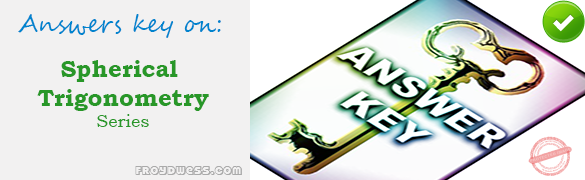# MCQ in Analytic Geometry: Parabola, Ellipse and Hyperbola Part 2 – Answers

(Last Updated On: February 5, 2020)Below are the answers key for the Multiple Choice Questions in Analytic Geometry: Parabola, Ellipse and Hyperbola – MCQ Part 2.

51. D. (-3, -3)

52. A. 4p

53. D. 8

54. A. 12

55. D. x = -4

56. D. Downward

57. D. Downward

58. C. 4x – 7 = 0

59. D. y2 – 6x + 3 = 0

60. D. x2 – 8x + 16y – 32 = 0

61. A. 10.67 sq. units

62. B. 10.7

63. A. 1.6

64. C. 3.6

65. B. 0.60

66. D. 0.866

67. B. 0.66

68. A. (2, 3)

69. C. 1.5

70. A. 15π sq. units

71. B. 62.8 square units

72. B. 6.047

73. C. 4

74. A. 12.5

75. A. 94,550,000 miles

76. D. 9×2 + 25y2 + 54x + 50y – 119 = 0

77. A. 4×2 + 3y2 = 12

78. D. 64x + 5y = 0

79. B. 4x – 3y – 20 = 0

80. A. 2

81. A. 2x – 3y = 0

82. C. -2

83. D. Circle

84. D. B2 – 4AC < 0

85. A. 3x + 5y -34 = 0

86. D. 3x + 4y – 12 = 0

87. B. 7x – 2y + 39 = 0

88. B. y = 4x – 1

89. A. 5x + 4y = 41

90. C. 2x + y – 9 = 0

91. D. y – 3 = 0

92. A. 3x – 4y = 0

93. C. 3r cos Ѳ + 4r sin Ѳ = 2

94. D. r2 = 8/(cos2 Ѳ + 2)

95. B. 10.14

96. D. y =√3 x

97. A. (2, 1, -1)

98. C. 15 sq. m.

99. C. 3

100. C.√101

### Online Questions and Answers in Analytic Geometry: Parabola, Ellipse and Hyperbola Series

Following is the list of practice exam test questions in this brand new series:

Analytic Geometry: Parabola, Ellipse and Hyperbola MCQs
PART 1: MCQs from Number 1 – 50                        Answer key: PART I
PART 2: MCQs from Number 51 – 100                        Answer key: PART I

P inoyBIX educates thousands of reviewers and students a day in preparation for their board examinations. Also provides professionals with materials for their lectures and practice exams. Help me go forward with the same spirit.

“Will you subscribe today via YOUTUBE?”

Subscribe

PinoyBIX Engineering. © 2014-2020 All Rights Reserved | How to Donate? |